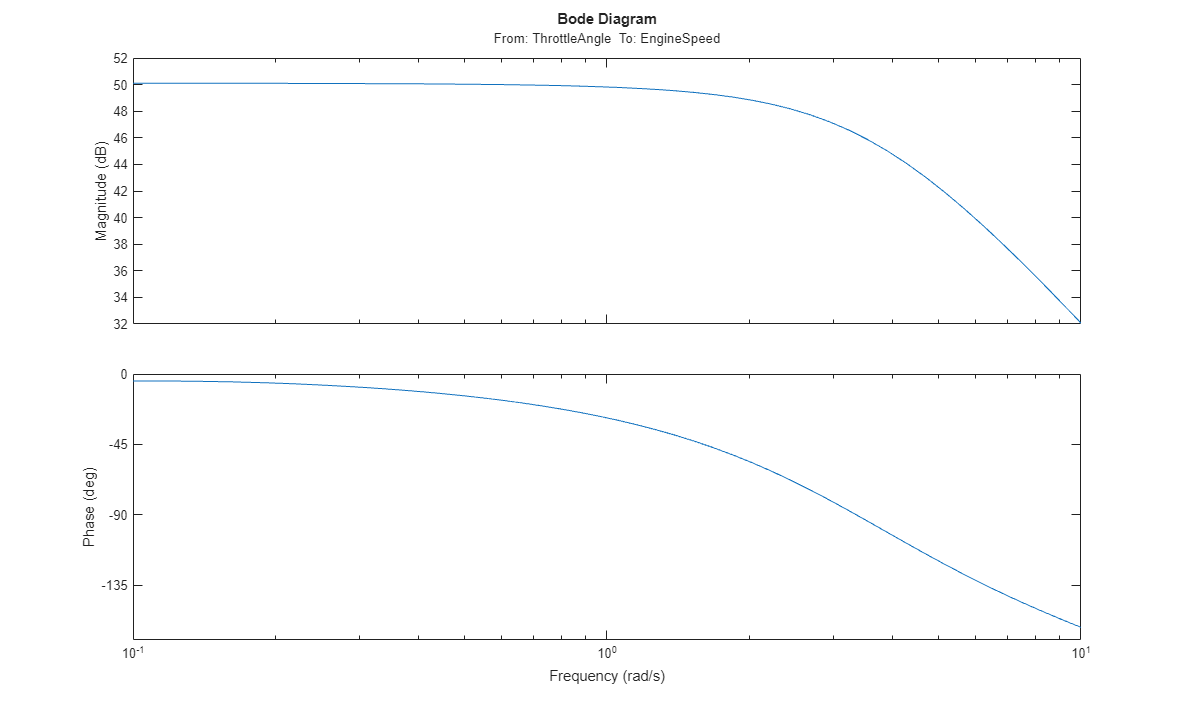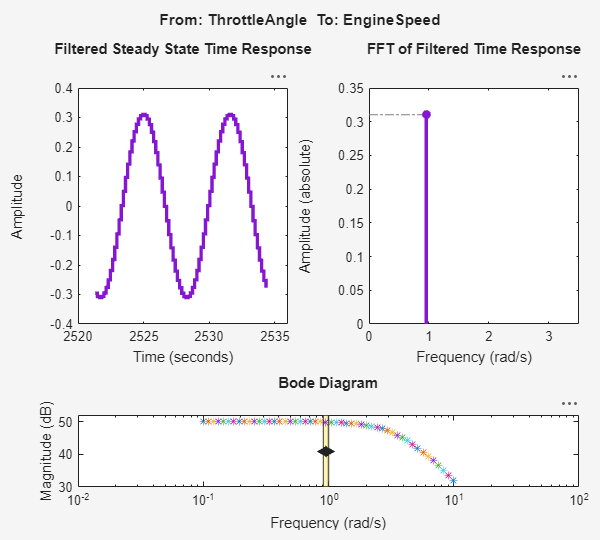# Frequency Response Estimation Using Simulation-Based Techniques

This example shows how to obtain the frequency response of Simulink® models when analytical block-by-block linearization does not provide an accurate answer due to event-based dynamics in the linearization path. Examples of such systems are models with triggered subsystems or models using pulse width modulation (PWM) input signals.

### Open the Model

Open the Simulink model for engine timing.

```mdl = 'scdengine'; open_system(mdl) ```Analytical block-by-block linearization of this model from the throttle angle to engine speed gives a zero linearization result due to the triggered Compression subsystem in the linearization path.

```io = getlinio(mdl); linsys = linearize(mdl,io) ```
```linsys = D = ThrottleAngl EngineSpeed 0 Static gain. ```

### Estimate Frequency Response Using Sinestream Input Signal

Sinestream input signals are the most reliable input signals for estimating an accurate frequency response of a Simulink model using the `frestimate` function. A sinestream signal is composed of individual sinusoidal signals that are appended to each other. The `frestimate` function simulates the model for each frequency in the sinestream input signal, as specified using the `Frequency` parameter, for the corresponding amount of periods, as specified using the `NumPeriods` parameter. After the simulation, `frestimate` uses the output signal to compute the response at each frequency. `frestimate` uses only the periods after the system reaches a steady state for that input frequency, that is, after a number of settling periods as specified using the `SettlingPeriods` parameter.

Create a sinestream signal with 50 logarithmically spaced distinct frequencies between 0.1 and 10 rad/s.

```in = frest.Sinestream('Frequency',logspace(-1,1,50),'Amplitude',1e-3) ```
``` The sinestream input signal: Frequency : [0.1 0.10985 0.12068 0.13257 ...] (rad/s) Amplitude : 0.001 SamplesPerPeriod : 40 NumPeriods : 4 RampPeriods : 0 FreqUnits (rad/s,Hz): rad/s SettlingPeriods : 1 ApplyFilteringInFRESTIMATE (on/off) : on SimulationOrder (Sequential/OneAtATime): Sequential ```

By default, each frequency in a sinestream input signal is simulated for four periods, that is, `NumPeriods` is `4` for all frequencies. Also, the end of the first period is specified to be where the system reaches steady state, that is, `SettlingPeriods` is `1` for all frequencies. Therefore, `frestimate` uses the last three periods of the output signals.

Using this sinestream input signal, perform the frequency response estimation using the `frestimate` function, and plot the resulting frequency response data.

```[sys,simout] = frestimate(mdl,io,in); bode(sys) ```You can inspect the estimation results using the Simulation Results Viewer. The viewer shows time-domain and frequency-domain simulation results for the selected frequencies and a summary bode plot where you can interactively switch between frequencies.

```frest.simView(simout,in,sys); ```You can use the viewer as a tool to diagnose issues that impact the accuracy of the frequency response estimation, such as:

• Excitation of nonlinearities

• Running into instabilities

### Estimate Frequency Response Using Chirp Input Signal

Another input signal you can use when estimating frequency response data from a Simulink model is a frequency chirp. A frequency chirp differs from a sinestream in that the frequency is instantaneously varied.

You can use chirp input signals to obtain faster frequency response estimation. However, the resulting frequency response can be less reliable than that obtained using sinestream inputs, since each frequency is not simulated long enough to drive the system to steady state at that frequency.

Create a chirp signal that sweeps between the frequencies 0.1 and 10 rad/s logarithmically.

```in_chirp = frest.Chirp('FreqRange',[0.1 10],'Amplitude',1e-3,... 'SweepMethod','logarithmic','NumSamples',3000); ```

Perform the frequency response estimation using the chirp signal.

```sys_chirp = frestimate(mdl,io,in_chirp); ```

Plot the results obtained from both the sinestream and chirp input signals together.

```bode(sys,sys_chirp,'r') ```### Estimate Frequency Response Using PRBS Input Signal

Another input signal you can use when estimating frequency response data from a Simulink model is a pseudorandom binary sequence (PRBS). A PRBS is a periodic, deterministic signal with white-noise-like properties that shifts between two values.

You can use PRBS input signals to obtain faster frequency response estimation with a higher frequency resolution than the chirp signal.

Create a PRBS signal with an order of `12` and one period in the signal. Using a single period produces a nonperiodic PRBS. The length of the generated PRBS is 4095 (2^12 - 1). To obtain an accurate frequency response estimation, the length of the PRBS must be sufficiently large. To ensure that the system is properly excited, specify the perturbation amplitude of the PRBS as `0.01`.

```in_PRBS = frest.PRBS('Order',12,'NumPeriods',1,'Amplitude',1e-2,'Ts',0.1,'UseWindow','off'); ```

Perform the frequency response estimation using the PRBS signal.

```sys_PRBS = frestimate(mdl,io,in_PRBS); ```

Plot the results obtained with both PRBS and sinestream input signals together.

```bode(sys_PRBS,sys,'r',{0.1 10}) ```Close the model.

```bdclose('scdengine') ```